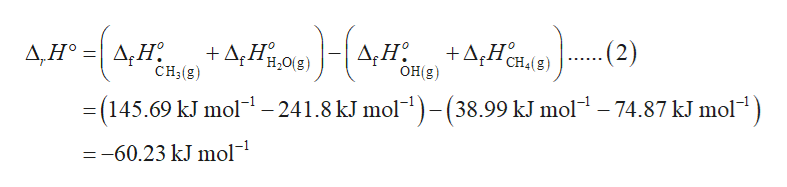# Calculate ?H° for each reaction.a. HO• + CH4 -> •CH3 + H2Ob. CH3OH + HBr -> CH3Br + H2O

Question
39 views

Calculate ?H° for each reaction.
a. HO• + CH4 -> •CH3 + H2O
b. CH3OH + HBr -> CH3Br + H2O

check_circle

Step 1

The chemical equation for the given chemical reaction is shown in equation (1).

Step 2

The standard enthalpy of a reaction (ΔrHo) is defined as the equation (2) where ΔfHoHO. is the standard enthalpy change of formation for hydroxyl radical, ΔfHoCH4(g), ΔfHoCH3(g), and ΔfHoH2O(g) is the standard enthalpy change for the formation of methane, methyl radical, and water vapor respectively. The values for standard enthalpy change for the formation of methyl radical, water vapor, hydroxyl radical, and methane are substituted in equation (2). The standard enthalpy of reaction is -60.23 kJ/mol.help_outlineImage Transcriptionclose+A,Hôn49) 2) +A;Hµ,0(3) A;H. Д,Н° %3D Δ. Η OH(g) H;O(g) CH4(g) ..... CH;(g) = (145.69 kJ mol– 241.8 kJ mol")-(38.99 kJ mol – 74.87 kJ mol") =-60.23 kJ mol- fullscreen
Step 3

The chemical equation for the given chemical reaction is shown in equation (...

### Want to see the full answer?

See Solution

#### Want to see this answer and more?

Solutions are written by subject experts who are available 24/7. Questions are typically answered within 1 hour.*

See Solution
*Response times may vary by subject and question.
Tagged in

### Chemical Thermodynamics